# Max-Cut

Max-Cut is the problem of finding cuts of maximum weight in graphs. It is one of the best-known and most studied problems of Combinatorial Optimization. Its description is as follows.

Our data is a weighted undirected graph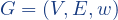, where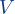is a set of nodes and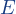is a set of edges, i.e., of unordered pairs of nodes. We denote an edge ofby the two nodes of the corresponding pair, thus, e.g.,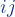is the edge havingand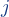as end-nodes. Every edgeofhas a weight, i.e., a real number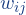.

For any partition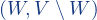of the node-set, we pick the edges of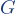having the two end-nodes in different sets of the partition. We call such a subset of edges a cut. Therefore, we have a cut for every partition of.  If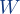is empty or if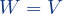, one of the two sets of the partition is empty, thus the corresponding cut has no edges. Ifhas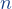nodes, the total number of partitions (including the degenerate partition with a single non-empty set) is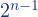therefore, these many are the different cuts of. The weight of a cut is the sum of the weights of its edges.

The Max-Cut Problem calls for a cut of maximum weight among all cuts of. Because an empty set is a legal cut of(whose weight is 0), the value of a maximum weight cut cannot be negative.

#### Why Max-Cut is a logoptimization problem?

How finding a cut of maximum weight in a graph can be seen as a logoptimization problem? If we assign a binary variable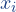to each nodeof, every assignment of the values 0 or 1 to these variables will correspond to a partition of. An edgebelongs to the cut associated with such a partition if and only if the values ofand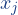differ. Therefore, we would need a function ofandthat has a value of 1 when the two variables have different values and 0 otherwise.

It is easy to verify that such a function can be expressed, using elementary arithmetic operations, as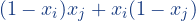. Then the weight of the cut can be expressed as the following polynomial of degree 2 in the variableswith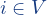: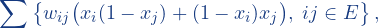that is the sum of the monomials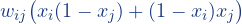for all edgesof. Maximizing such a sum is, evidently, a logoptimization problem.

#### Solving Max-Cut

The solution of Max-Cut to certified optimality is quite a difficult task for graphs of more than a few hundred nodes, unless the graph is very sparse. However, several techniques are available that produce good solutions to the problem along with a quality certificate.

One can generate a quality certificate by computing an upper bound to the problem, i.e., a value that is mathematically guaranteed not to be below the optimal value. Once an upper bound is at hand, it is possible to evaluate the quality of a given cut by computing the gap between its weight and the bound.

How difficult it is to find an upper bound? An easy way is to take the sum of the positive weights of alledges. Certainly, no cut can have a weight larger than this one. However, such an upper bound, which is very fast to compute, would generate, in general, horribly large gaps also for cuts very close to the optimum. Therefore, the challenge is to design efficient procedures that can compute upper bounds that are reasonably close to the optimal value.

#### SpeeDP

As said, fortunately, procedures are available that make it possible to find good cuts of very large graphs with a quality certified by a small gap. A very effective technique of this kind is based on the algorithm SpeeDP described in

L. Grippo, L. Palagi, M. Piacentini, V. Piccialli, and G. Rinaldi:
SpeeDP: An algorithm to compute SDP bounds for very large Max-Cut in­stan­ces.
Mathematical Programming 136 (2012), 353-373,

that reports the solution of instances of sizes up to a million nodes with gaps below 1%.

Our LOGOPTIMIZE server incorporates the SpeeDP algorithm and is able to handle very large instances of the problem, proving lower and upper bounds.